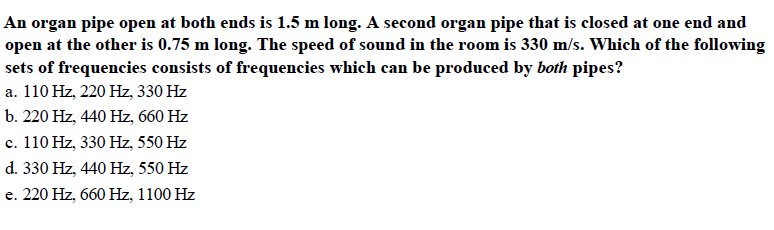An organ pipe open at both ends is 1.5 m long. A second organ pipe that is closed at one end andopen at the other is 0.75 m long. The speed of sound in the room is 330 m/s. Which of the followingsets of frequencies consists of frequencies which can be produced by both pipes?a. 110 Hz, 220 Hz, 330 Hzb. 220 Hz, 440 Hz, 660 Hzc. 110 Hz, 330 Hz, 550 Hzd. 330 Hz, 440 Hz, 550 Hze. 220 Hz, 660 Hz, 1100 Hz

Questionhelp_outlineImage TranscriptioncloseAn organ pipe open at both ends is 1.5 m long. A second organ pipe that is closed at one end and open at the other is 0.75 m long. The speed of sound in the room is 330 m/s. Which of the following sets of frequencies consists of frequencies which can be produced by both pipes? a. 110 Hz, 220 Hz, 330 Hz b. 220 Hz, 440 Hz, 660 Hz c. 110 Hz, 330 Hz, 550 Hz d. 330 Hz, 440 Hz, 550 Hz e. 220 Hz, 660 Hz, 1100 Hz fullscreen
Step 1

Given that the length of first pipe is 1.5m and it is open at both ends, the length of the second pipe is 0.75m and it is closed at one end. The frequency of different harmonics produced by an open pipe and a pipe with one end close are different. They are given below.

Step 2

In an open pipe, both odd and even harmonics are present. Substitute 1, 2, 3, 4, 5 etc. for n and the values of v and L to find the frequencies produced in the open pipe.

Step 3

In a pipe with one end closed, only odd harmonics are present. Substitute 1, 3, 5 etc. for n and the...

Want to see the full answer?

See Solution

Want to see this answer and more?

Our solutions are written by experts, many with advanced degrees, and available 24/7

See Solution
Tagged in

Wave Motion